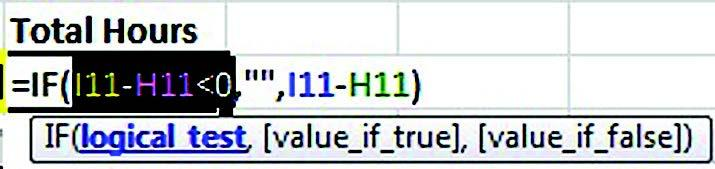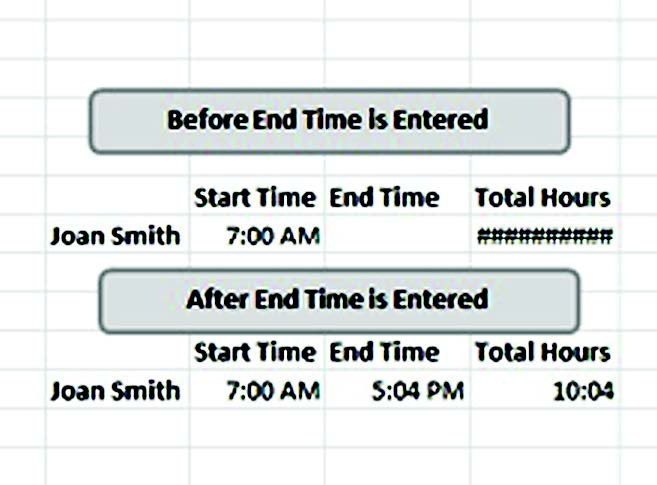You have probably been doing “if” logic ever since you were a toddler: If this is true, then this; otherwise, this.

IF statements are great for solving undesirable, but predicable, problems with error conditions. IF statements are good at solving problems with negative days or time. If you’ve ever tried to subtract a later date or time from an earlier one, you may have seen a very long string of # signs. That’s what happens in Excel when a cell is formatted for dates or times, but the result is a negative number.

For example, if you set up a simple time sheet with a formula that subtracts the end of workday time from the start time, you might get the # signs until you entered an end time.

To solve the problem, write a formula using an IF statement: IF(B1-A1<0,””,B1-A1). This results in a blank IF subtracting the start time from the end time as a negative number and simply does the math if it won’t.

Tip: Empty double quote marks (“”) mean “blank” to Excel in a formula. After the second comma, you can use a second IF statement. This is called nesting.

Here’s how IF statements work with this set of statements.

The first comma says THEN

The second comma says OTHERWISE

The OTHERWISE can be another IF

IF statements can be complicated.  Remember, all IF statements follow the same logic: If this is true, then this; otherwise, this. In Excel-speak, it is logical_test, value_­if_true, value_if_false. One way to unravel a complex IF statement is to use the pop-up tool tips that appear in edit mode in a formula and between the parentheses of a function.

You can navigate to the corresponding piece of the formula by clicking on the actual verbiage in the tool tip. Click on logical test and say, “If this is true.” Click on value if true and say “then this.” Click on value if false and say, “otherwise, this.”  This helps to dissect and understand IF statements other people wrote.Trace Precedents is another way to “reverse engineer” other people’s formulas. Look for the Trace Precedents button on the Formulas tab, Formula Auditing group. You should see arrows point to those cells if all elements of the formula are on the same page. If a component is on another spreadsheet, you’ll see a box and an arrow. Double-click on the arrowhead (not the box) to locate the other cells.

A new IF statement is now available in Excel 2007 and 2010, called IFERROR. If your formula may legitimately result in a #DIV/0 or an #N/A, you can have it appear blank by putting your whole function inside of an IFERROR function and specifying “” after the comma, like this =IFERROR(A1/B1,””) That means if A1/B1 will result in a #DIV/0, it will show a blank.